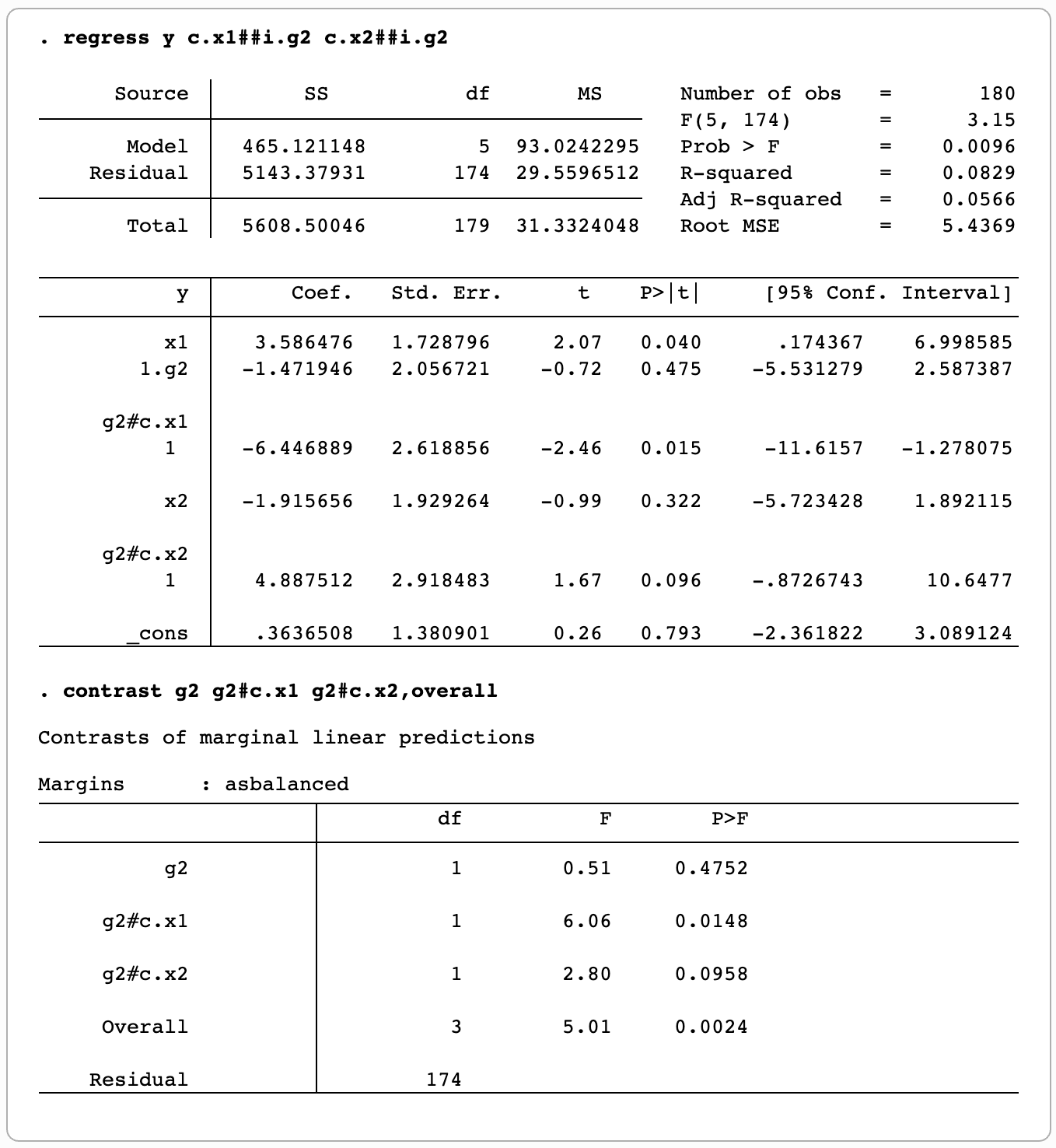# Stata command to perform Chow test

A Chow test is simply a test of whether the coefficients estimated over one group of the data are equal to the coefficients estimated over another.

I find two useful articles from Stata’s official website:

Suppose we do following regressions separately in two groups:

`regress y x1 x2 if group==1` and `regress y x1 x2 if group==2`

Then following commands will test the equality of coefficients on `x1` and `x2`:

`ge g2=(group==2)`
`regress y c.x1##i.g2 c.x2##i.g2`
`contrast g2 g2#c.x1 g2#c.x2, overall`

Stata’s official website gives an example of the output:In this example, to test the equality of coefficients on `x1` and `x2`, 6.06 and 2.80 are the F-stats that we are looking for.

This entry was posted in Stata. Bookmark the permalink.

### 5 Responses to Stata command to perform Chow test

1.Victor says:

Quick question. Can this be used when fixed effects are included in the regression of each group? Thanks.

•Kai Chen says:

I think it can. I did find the command quite flexible. Try i.factor##i.g2, it may work.

•Victor says:

Thanks. I used to add dummies and interact with every variable in order to test the difference in coefficients between groups, which was quite cumbersome.

2.Jodie says:

What if I just want to test the coefficient on x1?

3.Yuchen LUO says:

when do you want to use chow test in your research？ It seems to me that we only care about one or two variables and thus we usually do not fully interact the dummy variable of interest with all other variables. Many times, we look at the t-stats on the coefficients of the interaction term of interest and claim the whatever effect is stronger for a particular group.